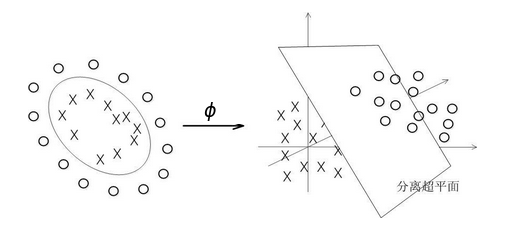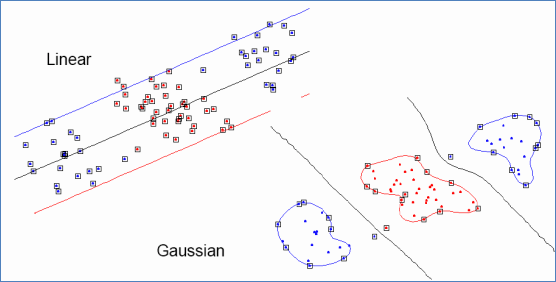# 机器学习----支持向量机（核函数）

#线性不可分 我们的SVM是找到一条分割直线，但是如果线性不可分怎么办？如下图：# 核函数

maxαipαi12i,j=1pyiyjαiαjxTixj

maxαipαi12i,j=1pyiyjαiαjϕ(xi)Tϕ(xj)

ϕ(xi)Tϕ(xj)=κ(xixj)

Sigmoid核 κ(xi,xj)=tank(βxTixj+θ)$\kappa(x_i,x_j)=tank(\beta x_i^{\mathop{T}}\cdot x_j+\theta)$ tanh为双曲正切函数，β>0,θ<0$\beta>0,\theta<0$